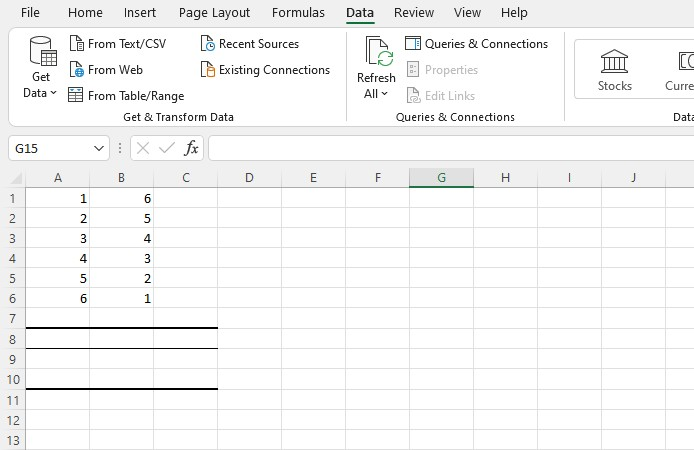# Calculating Correlation in Excel: Your How-To Guide

Microsoft Excel lets you do more than simply create spreadsheets — you can also use the software to calculate key functions, such as the relationship between two variables. Known as the correlation coefficient, this metric is useful for measuring the impact of one operation on another to inform business operations.

Not confident in your Excel skills? No problem. Here’s how to calculate — and understand — the correlation coefficient in Excel.

A correlation coefficient of +1 indicates a “perfect positive correlation”, which means that as variable X increases, variable Y increases at the same rate. A correlation value of -1, meanwhile, is a “perfect negative correlation”, which means that as variable X increases, variable Y decreases at the same rate. Correlation analysis may also return results anywhere between -1 and +1, which indicates that variables change at similar but not identical rates.

Correlation values can help businesses evaluate the impact of specific actions on other actions. For example, companies may find that as spending on social media marketing increases, so does customer engagement, indicating that more spending might make sense.

Or they may find that specific advertising campaigns result in a correlated decrease of customer engagement, in turn suggesting the need for a reevaluation of current efforts. The discovery that variables do not correlate can also be valuable; while common sense might suggest that a new function or feature in your product would correlate with increased engagement, it might have no measurable impact. Correlation analysis allows companies to view this relationship (or lack thereof) and make sound strategic decisions.

So how do you calculate the correction coefficient in Excel? Simple! Follow these steps:

### 1. Open Excel.

Step one: Open Excel and start a new worksheet for your correlated variable data. Enter the data points of your first variable in column A and your second variable in column B. You can add additional variables as well in columns C, D, E, etc. — Excel will provide a correlation coefficient for each one.

In the example below, we’ve entered six rows of data in column A and six in column B.

### 2. Install the Analysis Toolpak.

Next up? If you don’t have it, install the Excel Analysis Toolpak.

Select “File”, then “Options,” and you’ll see this screen: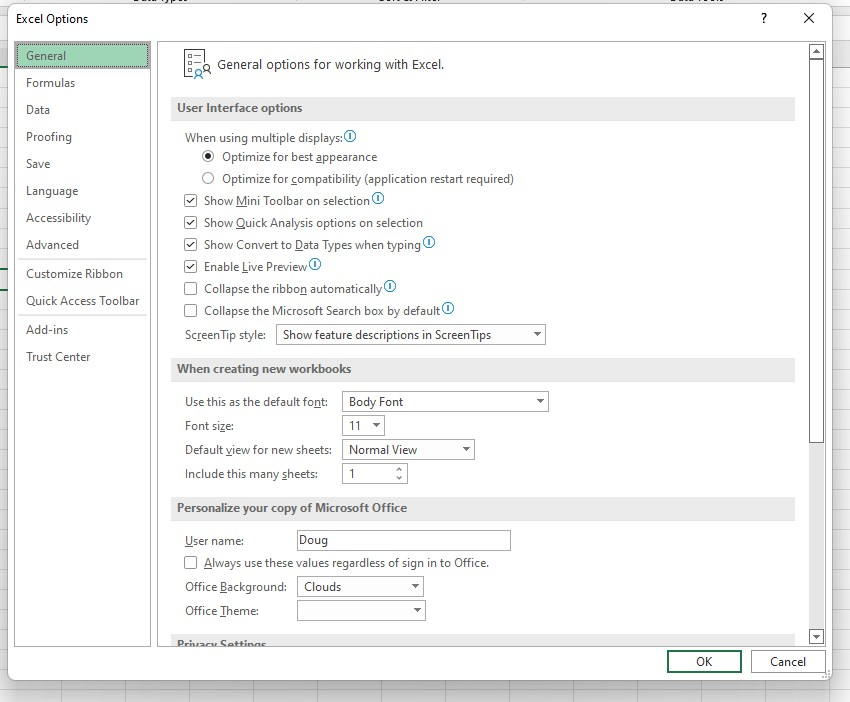Select “Add-Ins” and then click on “Go”.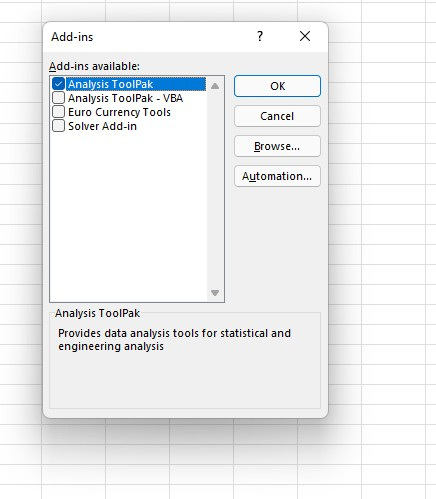Now, check the box that says “Analysis ToolPak” and click “Ok”.

### 3. Select “Data” from the top bar menu.

Once you have the ToolPak installed, select “Data” from the top Excel bar menu. This provides you with a submenu that contains a variety of analysis options for your data.

### 4. Select “Data Analysis” in the top right-hand corner.

Now, look for “Data Analysis” in the top right-hand corner and click on it to get this screen: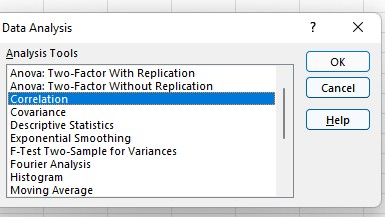### 5. Select Correlation.

Select Correlation from the menu and click “OK.”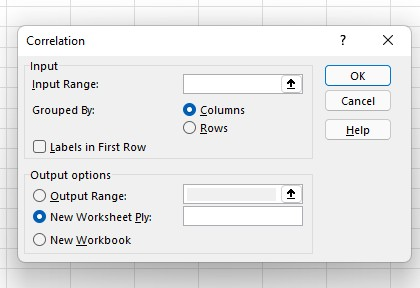### 6. Define your data range and output.

Now define your data range and output. You can simply left-click and drag your cursor across the data you want to select, and it will auto-populate in the Correlation box. Finally, select an output range for your correlation data — we’ve chosen A8. Then, click “Ok”.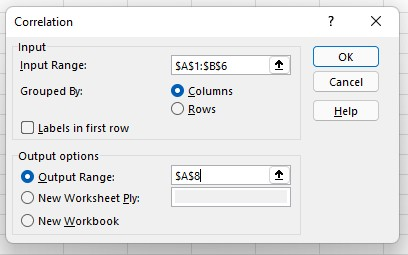### 7. Evaluate your correlation coefficient.

Your correlation results will now be displayed. In our example, values in column 1 and column 2 have a perfect negative correlation; as one goes up, the other goes down at the same rate.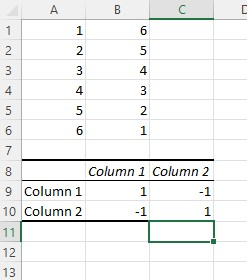## The Excel Correlation Matrix

Excel correlation results are also known as an Excel correlation matrix. In the example above, our two columns of data produced a perfect correction matrix of 1 and -1. But what happens if we produce a correlation matrix with a less ideal data set?

Here’s our data:

And here’s the matrix:

Cell C4 in the matrix gives us the correlation between Column 3 and Column 2, which is a very weak 0.01025, while Column 1 and Column 3 yield a stronger negative correlation of -0.17851. By far the strongest correlation, however, is between Column 1 and Column 2 at -0.66891.

So what does this mean in practice? Let’s say we were examining the impact of specific actions on the efficacy of a social media campaign, where Column 1 represents the number of visitors who click through on social advertisements and Columns 2 and 3 represent two different marketing taglines. The correlation matrix shows a strong negative correlation between Columns 1 and 2, which suggests that the Column 2 version of the tagline significantly decreased overall user engagement, while Column 3 drove only a slight decrease.

Regularly creating Excel matrices can help companies better understand the impact of one variable on another and determine what (if any) negative or positive effects may exist.

## The Excel Correlation Formula

If you prefer to enter the correlation formula yourself, that’s also an option. Here’s what it looks like:

X and Y are your measurements, ∑ is the sum, and the X and Y with the bars over them indicate the mean value of the measurements. You would calculate it as follows:

• Calculate the sum of variable X minus the mean of X.
• Calculate the sum of variable Y minus the mean of Y.
• Multiply those two results and set that number aside (this is the first result).
• Square the sum of X minus the mean of X. Square the sum of Y minus the mean of Y. Multiply those two numbers.
• Take the square root (this is the second result).
• Divide the first result by the second result.
• You get the correlation coefficient.

Easy, right? Yes and no. While plugging in the numbers isn’t complicated, it’s often more trouble than it’s worth to create and manage this formula. The built-in Excel Toolpak is often a simpler (and faster) way to pinpoint coefficients and discover key relationships.

## Correlation ≠ Not Causation

No article about correlation is complete without a mention that it does not equal causation. In other words, just because two variables rise or fall together doesn’t mean that one variable is the cause of the other variable’s increase or decrease.

Consider a few very strange examples.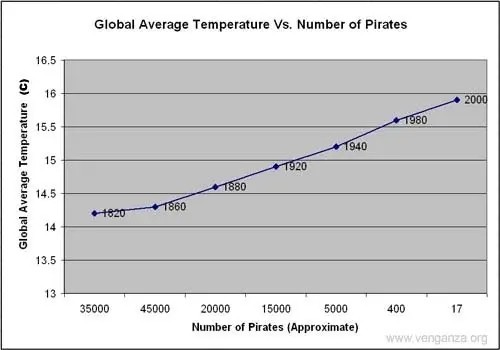This image shows a near-perfect negative correlation between the number of pirates and the global average temperature — as pirates became more scarce, the average temperature increased.

The problem? While these two variables are correlated, there’s no causal link between the two; higher temperatures did not reduce the pirate population and fewer pirates did not cause global warming.

While correlation is a powerful tool, it only indicates the direction of increase or decrease between two variables — not the cause of this increase or decrease. To discover causal links, companies must increase or decrease one variable and observe the impact. For example, if correlation shows that customer engagement goes up with social media spending, it’s worth opting for a slight increase in spending followed by a measurement of results. If more spending leads directly to increased engagement, the link is both correlated and causal. If not, there may be one (or more) factors that underpin the increase of both variables.

## Keeping Up with the Correlations

Excel correlations offer a solid starting point for marketing, sales, and spending strategy development, but they don’t tell the whole story. As a result, it’s worth using Excel’s built-in data analysis options to quickly evaluate the correlation between two variables and use this data as a jumping-off point for more in-depth analysis.